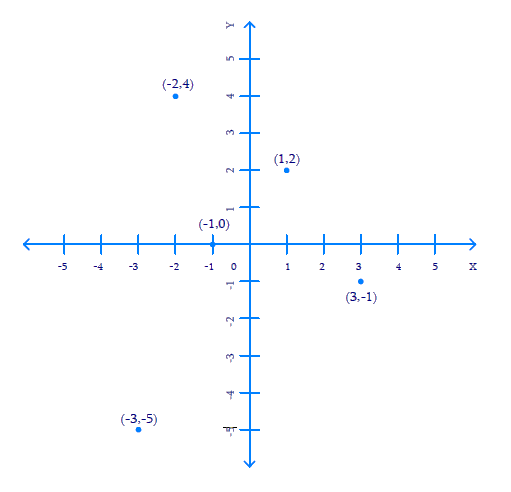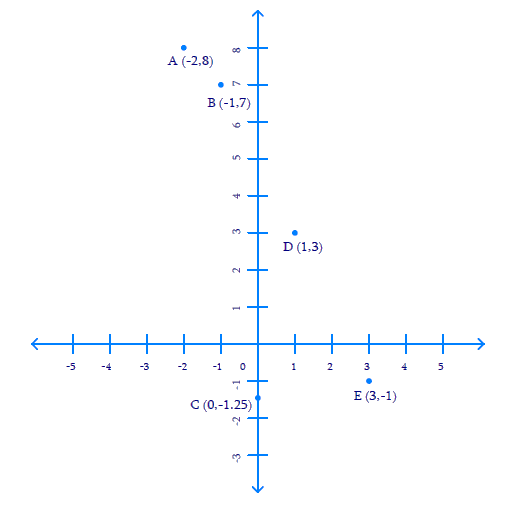# Exercise 3.3 Coordinate Geometry NCERT Solutions Class 9

## Chapter 3 Ex.3.3 Question 1

In which quadrant or on which axis do each of the points $$(–2, 4), (3, –1), (–1, 0), (1, 2)$$ and $$(–3, –5)$$ lie? Verify your answer by locating them on the Cartesian plane.

### Solution

Steps:

To determine the quadrant or axis of the points

$$(–2, 4), (3, –1), (–1, 0), (1, 2)$$

and $$(–3, –5)$$.

Plot the points

$$(–2, 4), (3, –1), (–1, 0), (1, 2)$$ and

$$(–3, –5)$$ on the graph, to getFrom the figure above, we can conclude that the points

• Point $$(–2, 4)$$ lie in $$2^\rm{nd}$$ quadrant
• Point $$(3, –1)$$ lie in $$4^\rm{th}$$ quadrant
• Point $$(–1, 0)$$ lie on the negative $$x-$$axis.
• Point $$(1, 2)$$ lie in $$1^\rm{st}$$ quadrant.
• Point $$(–3, –5)$$ lie in $$3^\rm{rd}$$quadrant.

## Chapter 3 Ex.3.3 Question 2

Plot the points ($$x, y$$) given in the following table on the plane, choosing suitable units of distance on the axes.

 $${x}$$ $$-2$$ $$-1$$ $$0$$ $$1$$ $$3$$ $$y$$ $$8$$ $$7$$ $$-1.25$$ $$3$$ $$-1$$

### Solution

Steps:

Given,

 $$x$$ $$-2$$ $$-1$$ $$0$$ $$1$$ $$3$$ $$y$$ $$8$$ $$7$$ $$-1.25$$ $$3$$ $$-1$$

Draw $$x$$-axis and $$y$$-axis as the co-ordinate axes and mark their point of intersection $$O$$ as the Origin ($$0, 0$$).

In order to plot the points given in the table provided above,

• Let us mark the first point as $$A\,(–2, 8)$$
• Take $$2$$ units on the negative $$x-$$axis and then $$8$$ units on positive $$y-$$axis.
• Let us mark the second point as $$B\,(–1, 7)$$
• Take $$-1$$ unit on negative $$x-$$axis and then $$7$$ units on positive $$y-$$axis.
• Let us mark the third point as $$C\,(0, –1.25),$$
• Take $$1.25$$ units below $$x-$$axis on negative $$y-$$axis.
• Let us mark the fourth point as $$D\,(1, 3)$$
• Take $$1$$ unit on positive $$x-$$axis and then $$3$$ units on positive $$y-$$axis.
• Let us mark the fifth point as $$E\,(3, –1)$$
• Take $$3$$ units on positive $$x-$$axis and then move $$-1$$ unit on negative $$x-$$axis.Instant doubt clearing with Cuemath Advanced Math Program